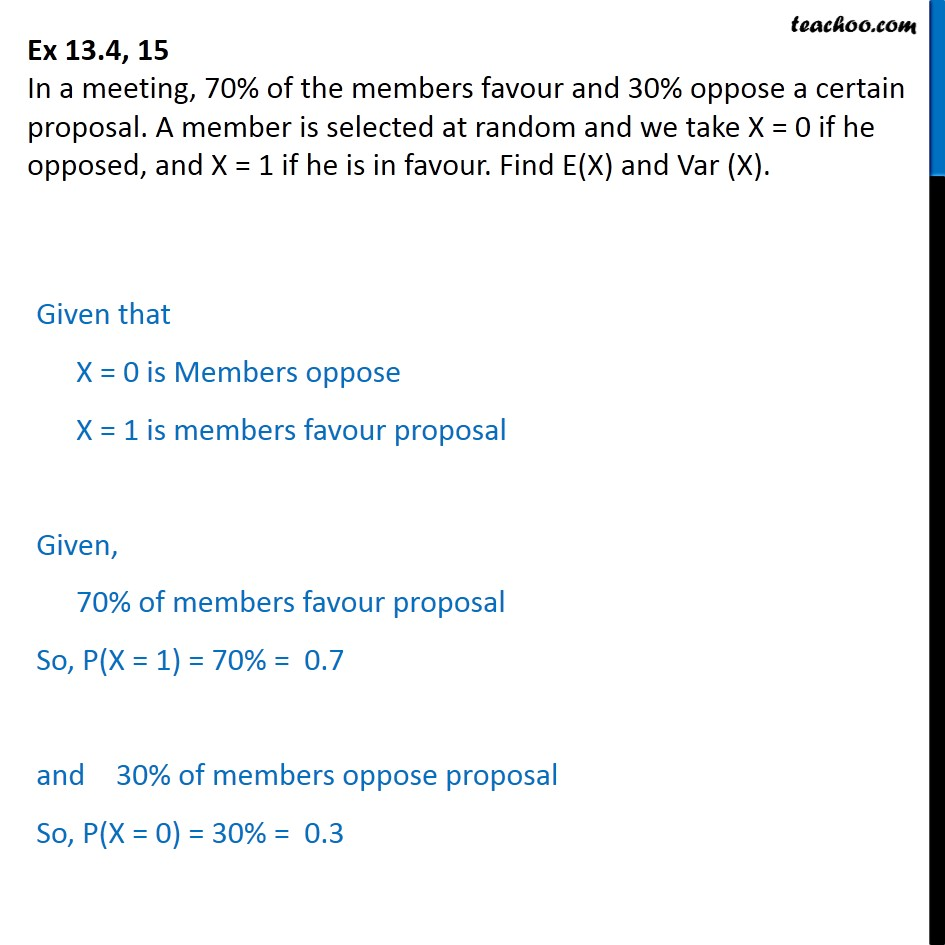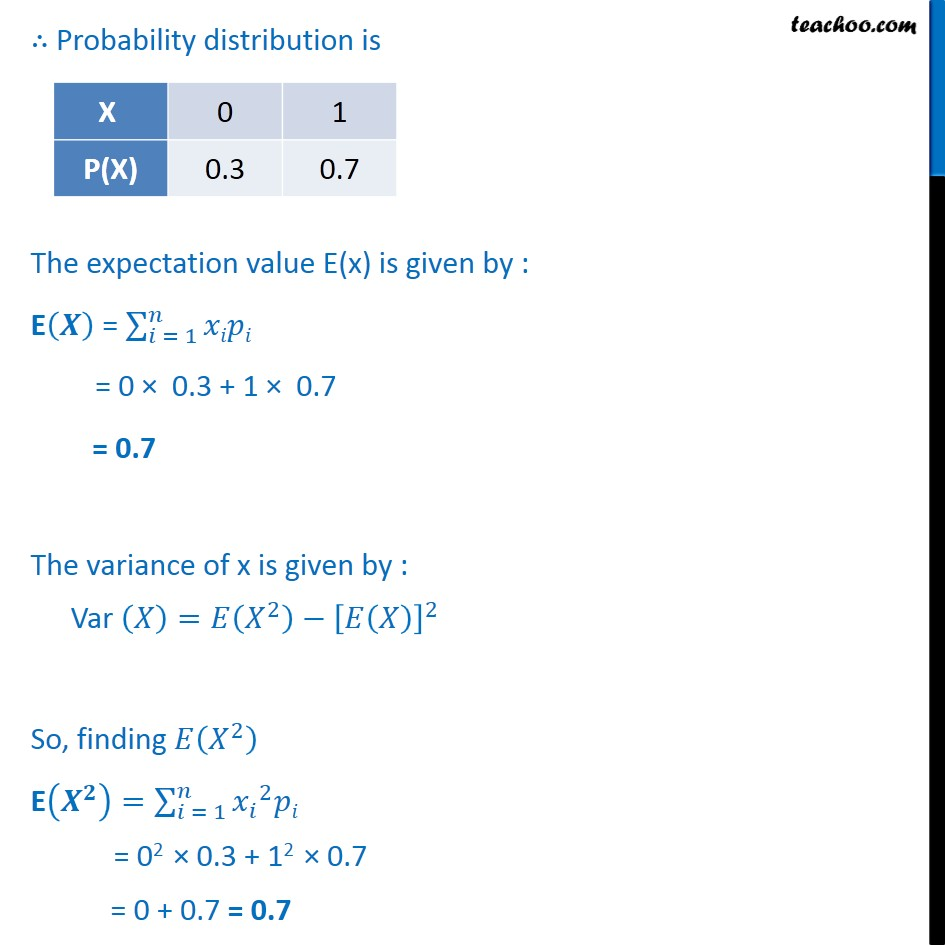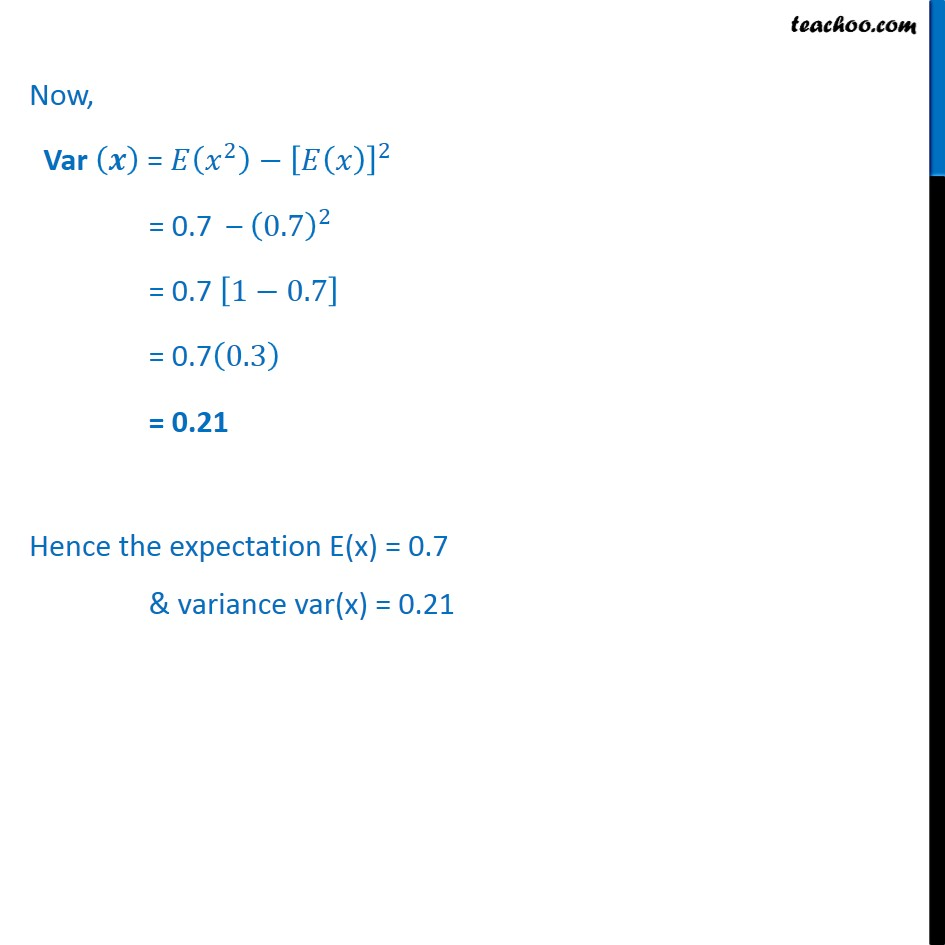Probability Distribution

Chapter 13 Class 12 Probability
Serial order wiseLearn in your speed, with individual attention - Teachoo Maths 1-on-1 Class

### Transcript

Question 15 In a meeting, 70% of the members favour and 30% oppose a certain proposal. A member is selected at random and we take X = 0 if he opposed, and X = 1 if he is in favour. Find E(X) and Var (X). Given that X = 0 is Members oppose X = 1 is members favour proposal Given, 70% of members favour proposal So, P(X = 1) = 70% = 0.7 and 30% of members oppose proposal So, P(X = 0) = 30% = 0.3 ∴ Probability distribution is The expectation value E(x) is given by : E 𝑿﷯ = 𝑖 = 1﷮𝑛﷮𝑥𝑖𝑝𝑖﷯ = 0 × 0.3 + 1 × 0.7 = 0.7 The variance of x is given by : Var 𝑋﷯=𝐸 𝑋﷮2﷯﷯− 𝐸 𝑋﷯﷯﷮2﷯ So, finding 𝐸 𝑋﷮2﷯﷯ E 𝑿﷮𝟐﷯﷯= 𝑖 = 1﷮𝑛﷮ 𝑥﷮𝑖﷯﷮2﷯𝑝𝑖﷯ = 02 × 0.3 + 12 × 0.7 = 0 + 0.7 = 0.7 Now, Var 𝒙﷯ = 𝐸 𝑥﷮2﷯﷯− 𝐸 𝑥﷯﷯﷮2﷯ = 0.7 – 0.7﷯﷮2﷯ = 0.7 1−0.7﷯ = 0.7 0.3﷯ = 0.21 Hence the expectation E(x) = 0.7 & variance var(x) = 0.21